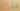ConditionalIsSequence

Misconception:

if c: A else: B is equivalent to if c: A if not c: B.Incorrect

If-else is equivalent to sequence of two ifs

Correct

If-else can behave differently from sequence of two ifs

CorrectionHere is what's right.

An if-else statement always executes only one of the two bodies:

if c:
a()
else:
b()

A sequence of two if-statements, however, may execute both bodies:

if c:
a()
if not c:
b()

Specifically, if evaluating c has side-effects, or if the value of c changes between the two tests (if c ..., if not c ...), then the two are different.

Example Causes for Differences

Condition with side effects:

def c():
print("c() called")  # side effect
return True

def if_else():  # prints once
if c():
a()
else:
b()

def two_ifs():  # prints twice
if c():
a()
if not c():
b()

Condition depending on state affected by a case (assume b() is an arbitrary function call):

def __init__(self):
self.state = False

def c(self):
return self.state  # accesses state that can be different for each call

def a(self):
self.state = False

def if_else(self):   # calls a()
self.state = True
if self.c():
self.a()
else:
self.b()

def two_ifs(self):  # calls a() and b()
self.state = True
if self.c():
self.a()
if not self.c():
self.b()

OriginWhere could this misconception come from?

If students previously learned a functional language, or if they remember functions defined by cases in mathematics, they may see a sequence of conditional statements as a declarative specification of different cases, and not as a sequence of execution steps.

SymptomsHow do you know your students might have this misconception?

This misconception can be detected by asking students to draw a control-flow graph of a sequence of if-statements.

ValueHow can you build on this misconception?

An occurrence of this misconception can be an opportunity for discussing the following aspects.

Functional vs. Imperative Programming

Students with this misconception may think in a more functional and less imperative way: they may see a sequence of if statements as a set of patterns to be matched and not really as a sequence of executions steps.

Elif with If Statements

When elif is used, the whole if statement looks like a multi-way branch:

if cond1:
a()
elif cond2:
b()
else:
c()

But due to potential side effects, the code above is not equivalent to:

if cond1:
a()
if not cond1:
if cond2:
b()
if not b:
c()

Python